# Retrieving the Value of the First Non-Blank Cell in a List in Microsoft Excel 2010

In this article, we will learn how to retrieve the value of the first Non-Blank cell. We use the Index function along with Match function in Microsoft Excel 2010 to get the desired result.

INDEX: The Index formula returns a value from the intersection between the row number and the column number in an Array. There are 2 syntaxes for the “INDEX” function.

1st Syntax of “INDEX” function: =INDEX (array, row_num, [column_num])

2nd Syntax of “INDEX” function:=INDEX (reference, row_num, [column_num], [area_num])

For example:- We have data in range A1:C5 in which column A contains Order Id, column B contains Unit Price, and column C contains Quantity.

• Write the formula in cell D2.
• =INDEX(A1:C5,2,3)
• Press Enter on your keyboard.
• The function will return 10, which means 10 is available in 2nd row and 3rd column.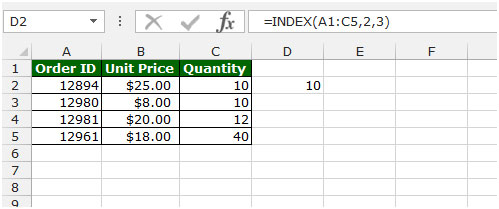MATCH: The Match formula returns the cell number where the value is found in a horizontal or vertical range.

Syntax of “MATCH” function:=MATCH(lookup_value,lookup_array,[match_type])

There are 3 match types. 1st is 1-less than, 2nd is 0-Exact match, 3rd is (-1)-greater than.

For example:- We have data in range A1:C5 in which Column A contains Order Id, column B contains Unit Price, and column C contains Quantity.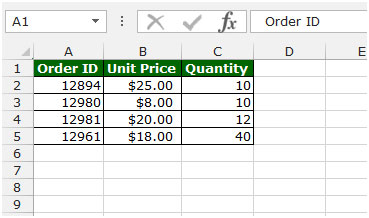• Write the formula in cell D2.
• =MATCH(12982,A2:A5,1)
• Press Enter on your keyboard.
• The function will return 4, which means 4th cell is matching as per given criteria.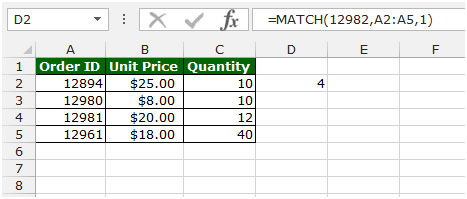Let’s take an example to understand how we can retrieve value of first non-blank cell.

We have data in range A1:A7 in which some cells are blank. Now, we want to retrieve the value of the first non-blank cell in range (A2:A7).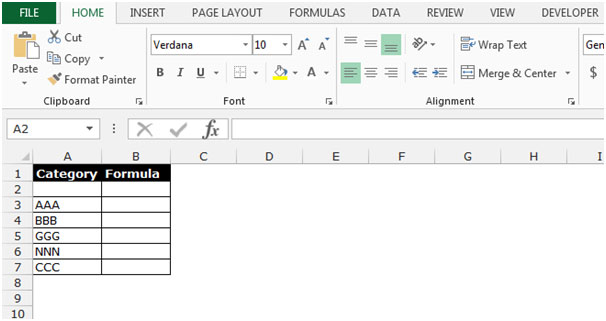• Write the formula in cell B2.
• =INDEX(A2:A7,MATCH(TRUE,A2:A7<>"",0))
• Press Ctrl+Shift+Enter on your keyboard.
• The function will return AAA, which means “AAA” is first non-blank cell’s value in the range.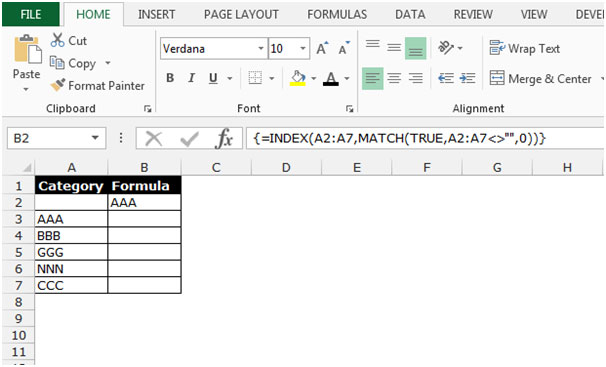Terms and Conditions of use

The applications/code on this site are distributed as is and without warranties or liability. In no event shall the owner of the copyrights, or the authors of the applications/code be liable for any loss of profit, any problems or any damage resulting from the use or evaluation of the applications/code.